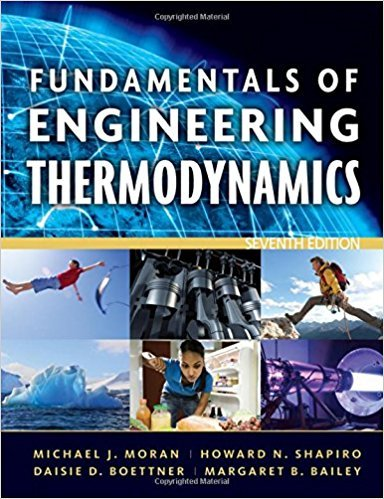×
×

# Exploring Energy ConceptsWhat is the overall change inISBN: 9780470495902 50

## Solution for problem 5P Chapter 2

Fundamentals of Engineering Thermodynamics | 7th Edition

• Textbook Solutions
• 2901 Step-by-step solutions solved by professors and subject experts
• Get 24/7 help from StudySoup virtual teaching assistantsFundamentals of Engineering Thermodynamics | 7th Edition

4 5 1 405 Reviews
11
5
Problem 5P

Exploring Energy Concepts

What is the overall change in potential energy, in ft · lbf and Btu, of an automobile weighing 2500 lbf in a drive from San Diego, CA to Santa Fe, NM? Take g constant.

Step-by-Step Solution:

Step 1 of 3:

Consider an automobile is traveling from San Diego, California to Santa Fe, New Mexico. The elevation above the sea level of Santa Fe is very much greater. Hence the potential energy of the vehicle increases as the automobile travel. Assume that the acceleration due to gravity is constant. We are going to find the change in potential energy in ft · lbf and Btu.

The weight of the vehicle W = 2,500 lbf

The difference in the elevation between the two cities (h2 - h1)=  7,140 ft (approximately)

Step 2 of 3:

The change in potential energy of the automobileThis is the change in potential energy of the automobile in ft · lbf.

Step 3 of 3

##### ISBN: 9780470495902

Unlock Textbook Solution